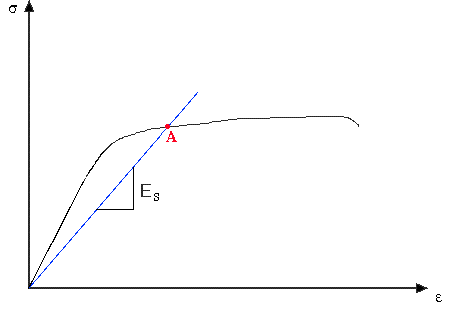# Strength and Stiffness Characteristics

 Secant Modulus: Secant modulus is the slope of a line drawn from the origin of the stress-strain diagram and intersecting the curve at the point of interest. Therefore, the secant modulus can take different values depending on the location of intersect. The figure below shows how the secant modulus is obtaind at point A on the curve. The secant modulus can be expressed as a percentage of the Young's Modulus (e.g., 0.7E or 0.85E), and it is used to describe the stiffness of a material in the inelastic region of the stress-strain diagram. Secant modulus is commonly denoted by Es.